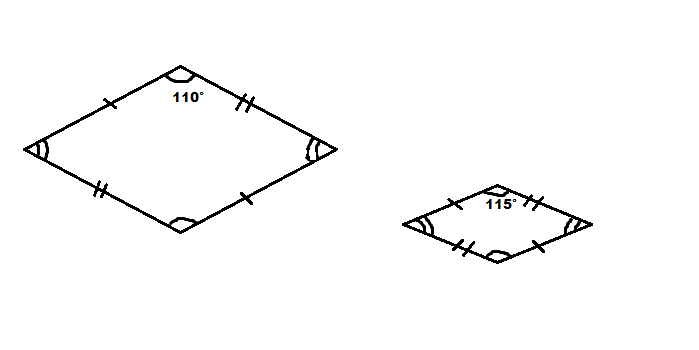## Example Questions

### Example Question #1 : How To Find If Rhombuses Are Similar

A quadrilateral ABCD has diagonals that are perpendicular bisectors of one another. Which of the following classifications must apply to quadrilateral ABCD?

I. parallelogram

II. rhombus

III. square

I, II, and III

I and II only

I and III only

II and III only

I and II only

Explanation:

If the diagonals of a quadrilateral are perpendicular bisectors of one another, then the quadrilateral must be a rhombus, but not necessarily a square. Since all rhombi are also parallelograms, quadrilateral ABCD must be both a rhombus and parallelogram.

### Example Question #2 : How To Find If Rhombuses Are SimilarAre the two rhombuses in the above picture similar?

Not enough information to decide.

No

Yes

Maybe